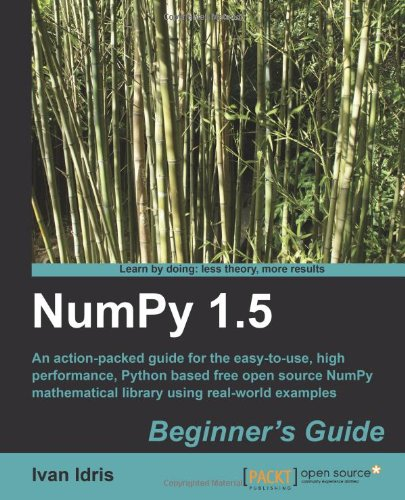# NumPy 1.5 Beginner’s Guide

• Length: 234 pages
• Edition: 1
• Publisher:
• Publication Date: 2011-12-02
• ISBN-10: 1849515301
• ISBN-13: 9781849515306
Description

An action packed guide for the easy-to-use, high performance, free open source NumPy mathematical library using real world examples

• The first and only book that truly explores NumPy practically
• Perform high performance calculations with clean and efficient NumPy code
• Analyze large data sets with statistical functions
• Execute complex linear algebra and mathematical computations

In Detail

In today’s world of science and technology, the hype is all about speed and flexibility. When it comes to scientific computing, NumPy is on the top of the list. NumPy is the fundamental package needed for scientific computing with Python. NumPy will give you both speed and high productivity. Save thousands of dollars on expensive software, while keeping all the flexibility and power of your favourite programming language.

NumPy 1.5 Beginner’s Guide will teach you about NumPy from scratch. It includes everything from installation, functions, matrices, and modules to testing, all explained with appropriate examples.

Numpy 1.5 Beginner’s Guide will teach you about installing and using NumPy and related concepts.

This book will give you a solid foundation in NumPy arrays and universal functions. At the end of the book, we will explore related scientific computing projects such as Matplotlib for plotting and the SciPy project through examples.

NumPy 1.5 Beginner’s Guide will help you be productive with NumPy and write clean and fast code.

What you will learn from this book

• Installing NumPy
• Learn to load arrays from files and write arrays to files
• Work with universal functions
• Create NumPy matrices
• Use basic modules that NumPy offers
• Write unit tests for NumPy code
• Plot mathematical NumPy results with Matplotlib
• Integrate with Scipy, a high level Python scientific computing framework built on top of NumPy

Approach

The book is written in beginner’s guide style with each aspect of NumPy demonstrated by real world examples. There is appropriate explained code with the required screenshots thrown in for the novice.

Who this book is written for

This book is for the programmer, scientist or engineer, who has basic Python knowledge and would like to be able to do numerical computations with Python.